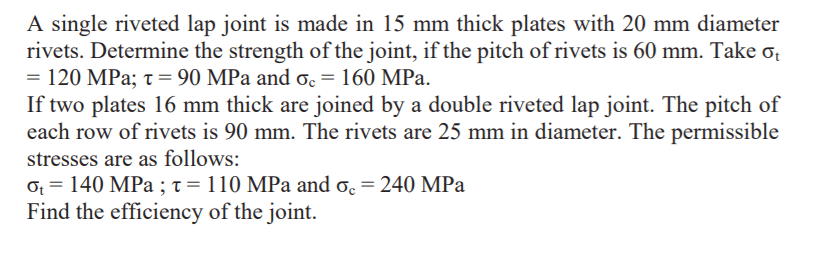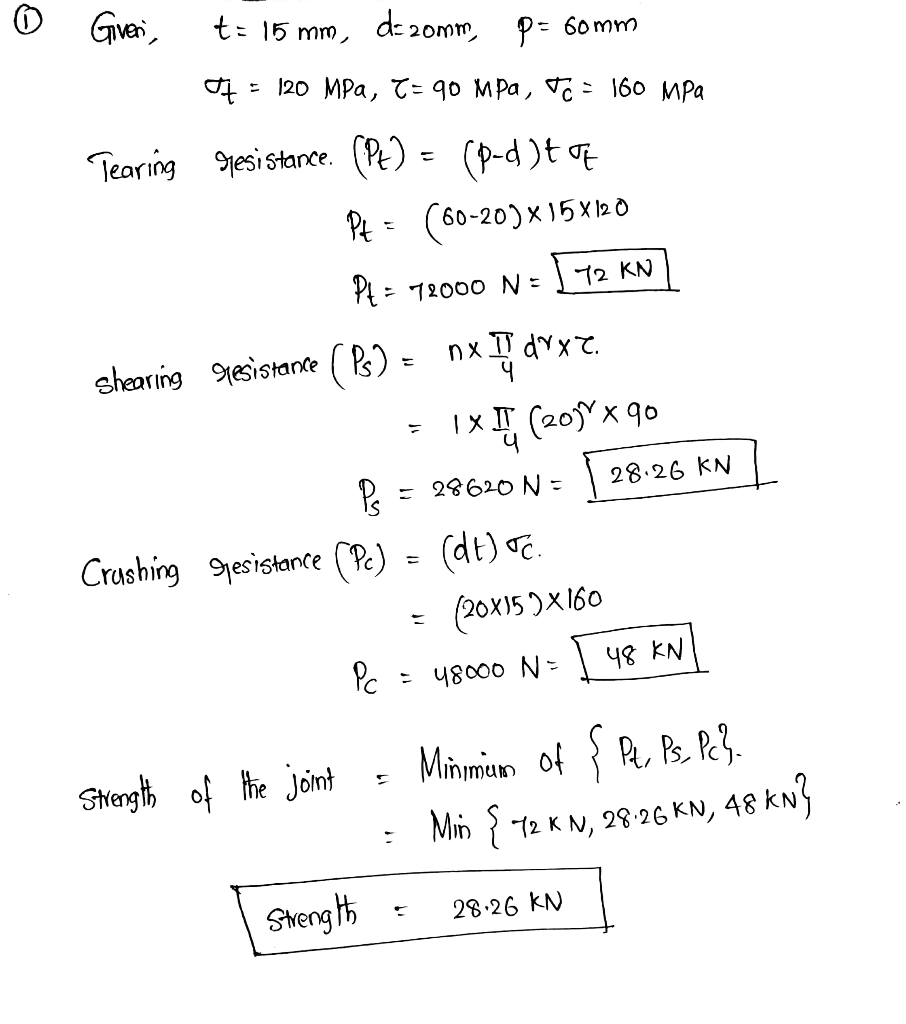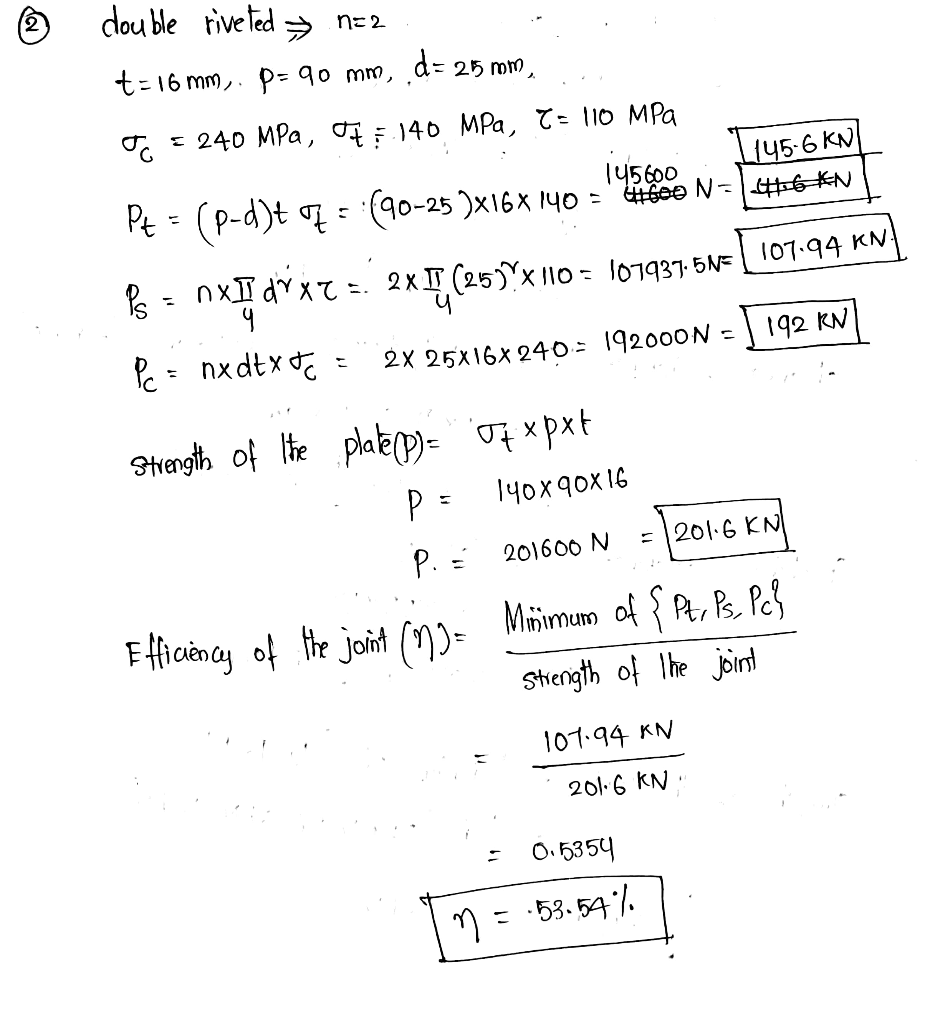# A single riveted lap joint is made in 15 mm thick plates with 20 mm diameter rivets. Determine the strength of the joint, if the pitch of rivets is 60 mm. Take  σt = 120 MPa; τ=90 MPa and σc=160 MPa. If two plates 16 mm thick are joined by a double riveted lap joint. The pitch of each row of rivets is 90 mm. The rivets are 25 mm in diameter. The permissible stresses are as follows: σt = 140 MPa ; τ = 110 MPa and σc = 240 MPa Find the efficiency of the joint.

Question-AnswerCategory: Machine DesignA single riveted lap joint is made in 15 mm thick plates with 20 mm diameter rivets. Determine the strength of the joint, if the pitch of rivets is 60 mm. Take  σt = 120 MPa; τ=90 MPa and σc=160 MPa. If two plates 16 mm thick are joined by a double riveted lap joint. The pitch of each row of rivets is 90 mm. The rivets are 25 mm in diameter. The permissible stresses are as follows: σt = 140 MPa ; τ = 110 MPa and σc = 240 MPa Find the efficiency of the joint.

A single riveted lap joint is made in 15 mm thick plates with 20 mm diameter rivets. Determine the strength of the joint, if the pitch of rivets is 60 mm. Take  σt = 120 MPa; τ=90 MPa and σc=160 MPa. If two plates 16 mm thick are joined by a double riveted lap joint. The pitch of each row of rivets is 90 mm. The rivets are 25 mm in diameter. The permissible stresses are as follows: σt = 140 MPa ; τ = 110 MPa and σc = 240 MPa Find the efficiency of the joint.